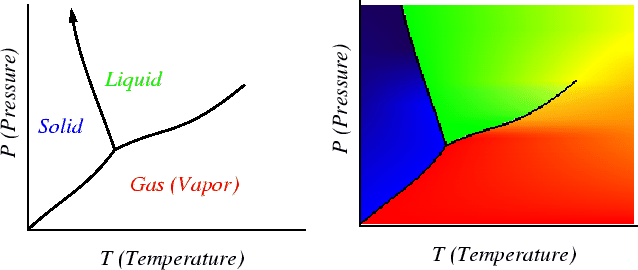Next: Solution Thermodynamics Up: Continuum Thermodynamics Previous: Gibbs-Duhem Equation

## The Relation between Gibb's Free Energy and Equilibrium Phase Diagrams

The equality of the molar Gibbs free energy can be graphically depicted in phase diagrams. It is very important for you to understand how the Gibbs free energy is related to phase diagrams. This is very very important.

Here is a single component phase diagram:A phase diagram is a map that tells you what phases will be stable at what conditions. You must understand and be able to explain how these diagrams follow from plots of free energy curves--this involves using intersections of the free energy curves and the common tangent construction.

W. Craig Carter 2002-09-05